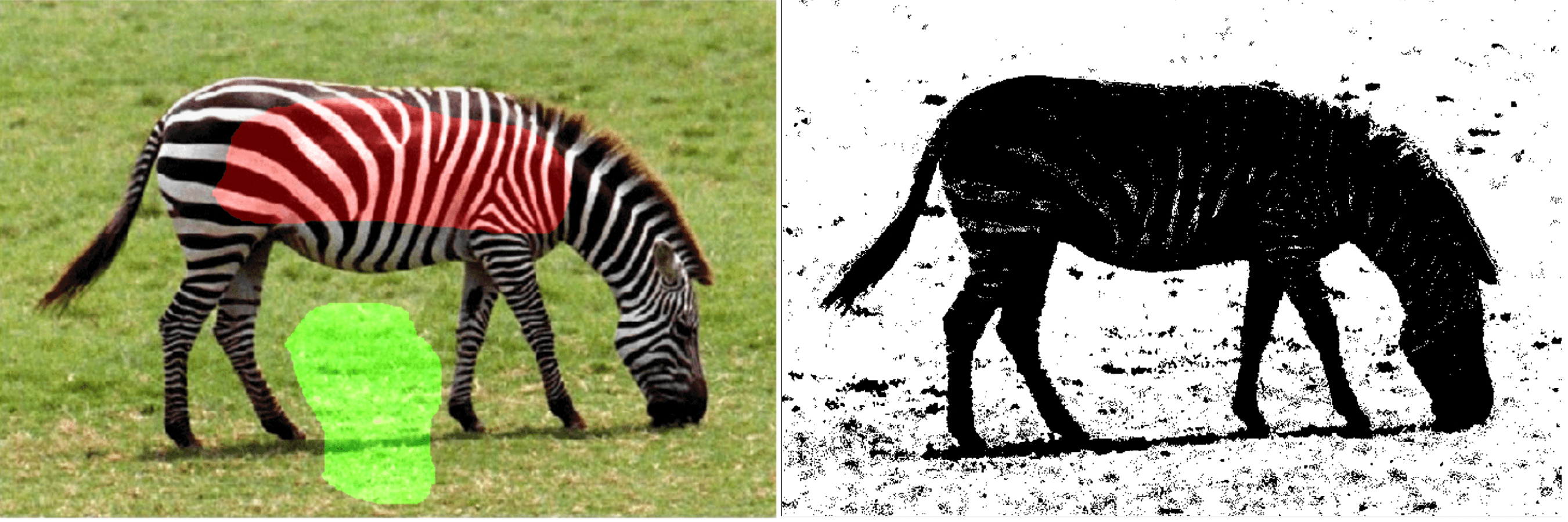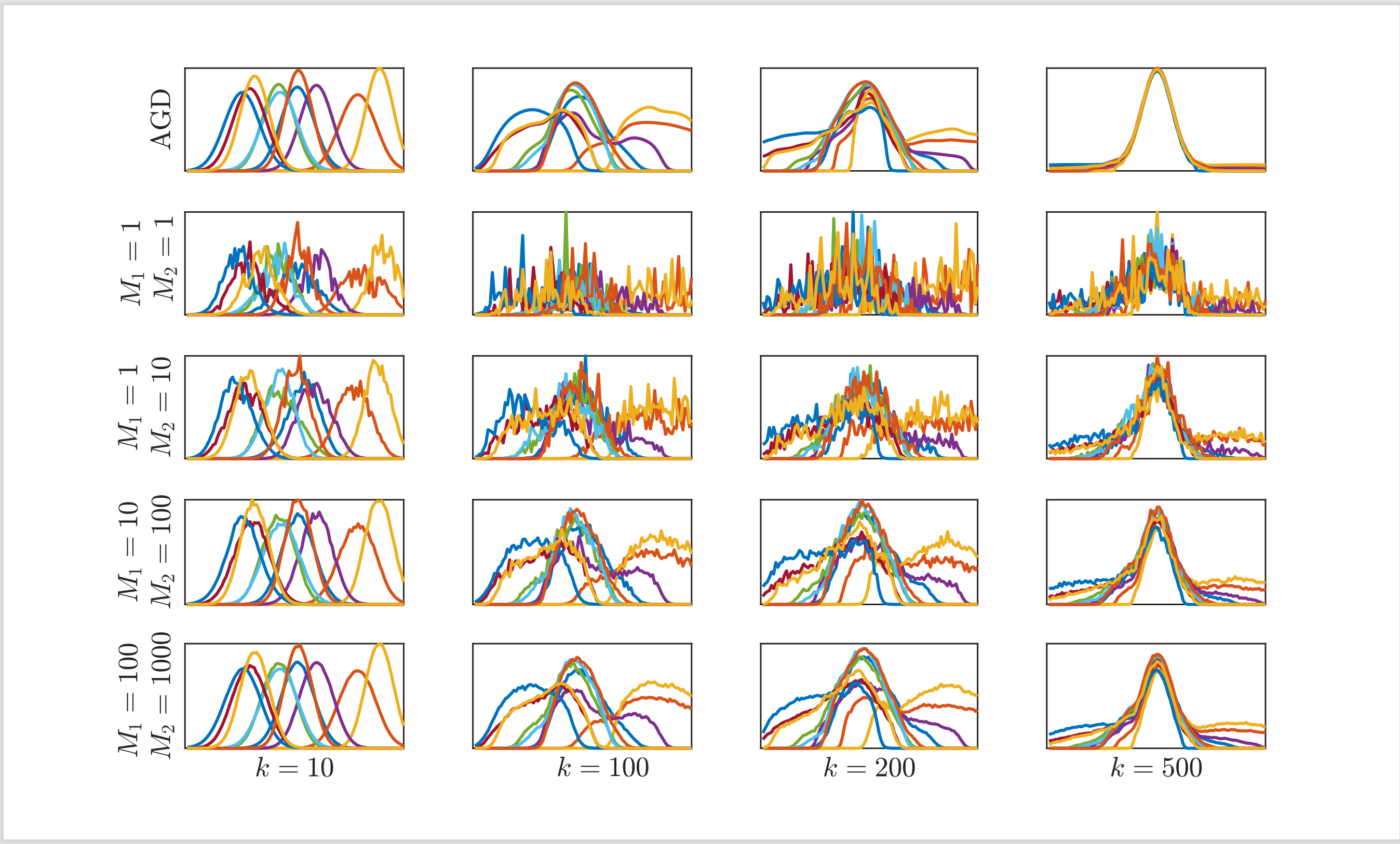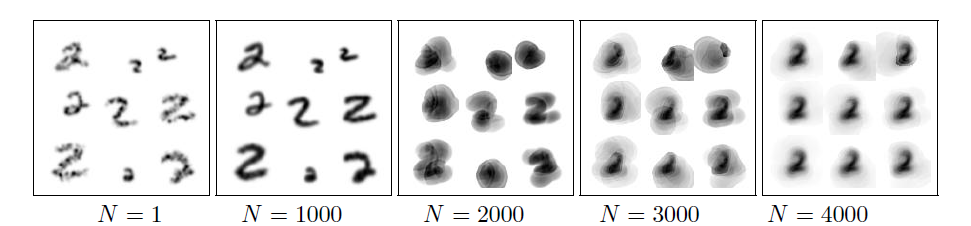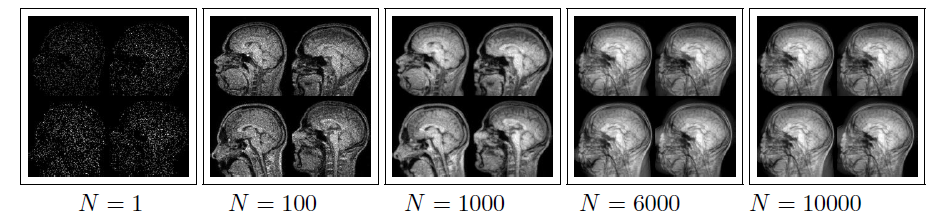# EF3 – Model-Based Imaging

## Optimal Transport for Imaging

Pavel Dvurechensky, Michael Hintermüller, Vladimir Spokoiny

Project Members

Roman Alexander Krawtschenko (HU)

Project Duration

01.07.2019 – 30.06.2022

Located at

HU Berlin

### Description

Optimal transport (OT) distances between probability measures or histograms capture the geometric nature of complex imaging data and play an increasing role in imaging, e.g. in image retrieval, clustering, classification, segmentation, etc. Project aims at developing theoretically and computationally solid procedures for imaging, based on the OT approach. Two particular applications are considered: image segmentation and image classification with the goal of analyzing medical images.

General introduction to OT

Given a basis space and a transportation cost function, the OT approach defines a distance between two objects as a distance between two probability measures on a basis space. Consider an example of two gray-scale images. In this case, the basis space can be defined as the pixel grid of this image. Then, for each pixel, the intensity can be seen as a mass situated at this pixel. Normalizing the intensity of each pixel dividing it by the total mass of the image, one obtains a discrete probability measure (or histogram) on the space of pixel grid. Then, the squared Euclidean distance between two pixels can be taken as a cost of transportation of a unit mass between these two pixels. Given two images, which are now modeled as probability measures, the goal is to transport the first image to the second. To do so, a transportation plan  is defined as a measure on the direct product of the basis pixel space by itself, i.e. a matrix with elements summing up to 1. Each row of this matrix corresponds to a pixel of the first image and tells what portion of mass is transported from this pixel to each pixel of the second image, see Figure.At the same time, each column of this matrix corresponds to a pixel of the second image and tells what portion of mass is transported to this pixel from each pixel of the first image. Note that this definition is symmetric as the same transportation plan can be used to transport the second image to the first one.

Given squared euclidean distance as transportation cost (i.e., for each pair of pixels, the cost of transporting a unit of mass from a pixel in the first image to a pixel in the second) and transportation plan (i.e., for each pair of pixels, the mass which is transported from a pixel in the first image to a pixel in the second), one can calculate the total cost of transportation of the first image to the second by summing individual costs for each pair of pixels and finding the total cost. Now the the transportation plan can be varied in order to find the minimum possible total cost of transportation. The square root of this minimum transportation cost is called Monge-Kantorovich or Wasserstein distance on the space of probability measures. This is a particular case of optimal transport distance. Optimal transport theory proves that this minimum is attained and is indeed a distance which satisfies all the distance axioms. Above we mainly considered an example of images. But, this distance is defined between general probability measures.

Image segmentation

We are building upon the approach of , where it is proposed to characterize image segments by their histograms of features. Consider, for example, gray-scale images with the intensity divided into several intervals, called bins. Then, given a segment of an image, the histogram is the vector with dimension equal to the number of bins and components equal to the proportion of the pixels having intensity falling into the respective bin. Since the proportion is evaluated, the histogram vector is a discrete probability distribution. Then one can define a data fitting term, which, when minimized, gives the segmentation of the image. This is done in a supervised setting by first taking a fraction of pixels which are known to belong to the object in the image and constructing a histogram of this object subregion. Then the goal is to find all the pixels from the object by minimizing the OT distance between histogram of the whole object region and the histogram of the subregion. A total variation regularization is used along with this data fitting term, to penalize large perimeter of the segmented object. The goal of this subproject is to extend this framework in several directions. The first one is allowing continuous image space, which gives resolution-independent algorithms using infinite-dimensional optimization, e.g. by Semi-Smooth Newton Method. Next, generalized total variation regularization is applied to allow for space-dependent regularization which is adaptive to local features in the image and is able, e.g. to segment thin lines. Further direction is to use unbalanced optimal transport in order to make transition to unsupervised setting.

Image classification

In this subproject OT barycenters are used to define typical object in a dataset of random images. For example, in the classification problem with two classes, one can define average object for the first class and for the second. Then, for a new object, classification is made using the closest average object. The challenge consists in the appropriate definition of this average for complex objects such as images. It was empirically shown in  that OT barycenter, defined as a minimizer of sum of OT distances to all the objects in the set, gives a reasonable average object. Yet, finding this minimizer is computationally intensive since one more level of optimization is added and it is required to find minimum of sum of minimums as OT distance is itself given by minimization problem. Statistical inference questions need also to be addressed. For example, is it true that as the sample size grows, the barycenter converges to the true average object. This subproject is aimed to propose computationally efficient numerical methods for finding Wasserstein barycenter based on the paradigm of distributed optimization dealing with distributed datasets and allowing only local communications between nodes in the computational network. Another goal is to study statistical inference via OT, e.g. establishing central limit theorem for the barycenter of random sample of measures.

References

1. Kantorovich, L.: On the translocation of masses.Doklady Acad. Sci. USSR (N.S.), 37:199–201, 1942.
2. Papadakis, N., Rabin, J.: Convex histogram-based joint image segmentation with regularized optimaltransport cost.Journal of Mathematical Imaging and Vision, 59(2):161–186, 2017.
3. Cuturi, M., Doucet, A.: Fast computation of Wasserstein barycenters. In: Xing,E.P., Jebara, T. (eds.) Proceedings of the 31st International Conference on Machine Learning. Proceedings of Machine Learning Research, vol. 32, pp. 685–693, 2014.

Project Webpages

Related Publications

1. Kroshnin, A., Tupitsa, N., Dvinskikh, D., Dvurechensky, P., Gasnikov, A., and Uribe, C. On the
complexity of approximating Wasserstein barycenters. In Proceedings of the 36th International Conference on
Machine Learning, K. Chaudhuri and R. Salakhutdinov, Eds.,
vol. 97 of Proceedings of Machine Learning Research, pp. 3530-3540 (2019). http://proceedings.mlr.press/v97/kroshnin19a.html
2. Dvinskikh, D., Gorbunov, E., Gasnikov, A., Dvurechensky, P., and Uribe, C. A. On primal and
dual approaches for distributed stochastic convex optimization over networks. In 2019 IEEE 58th Conference on
Decision and Control (CDC) (2019), pp. 7435-7440. arXiv:1903.09844
3. Guminov, S., Dvurechensky, P., Tupitsa, N., and Gasnikov, A. On a combination of alternating minimization and Nesterov’s momentum. In Proceedings of the 38th International Conference on Machine Learning, M. Meila and T. Zhang, Eds., vol. 139 of Proceedings of Machine Learning Research, pages 3886–3898 (2021). https://proceedings.mlr.press/v139/guminov21a.html
4. Tupitsa, N., Dvurechensky, P., Gasnikov, A., and Guminov, S. Alternating minimization methods for
strongly convex optimization. Journal of Inverse and Ill-posed Problems (2021). arXiv:1911.08987
5. Dvurechensky, P., Gasnikov, A., Ostroukhov, P., Uribe, C. A., and Ivanova, A. Near-optimal tensor
methods for minimizing the gradient norm of convex function. arXiv:1912.03381 (2019). Submitted to Optimization Methods and Software.
6. Tupitsa, N., Dvurechensky, P., Gasnikov, A., and Uribe, C. A. Multimarginal optimal transport by
accelerated gradient descent. In 2020 IEEE 59th Conference on Decision and Control (CDC) (2020). arXiv:2004.02294
7. Krawtschenko, R., Uribe, C. A., Gasnikov, A., and Dvurechensky, P. Distributed optimization with
quantization for computing Wasserstein barycenters. http://10.20347/WIAS.PREPRINT.2782, arXiv:2010.14325 (2020).
8. Bachoc, F., Suvorikova, A., Ginsbourger, D., Loubes, J.-M., Spokoiny, V. Gaussian processes with multidimensional distribution inputs via optimal transport and Hilbertian embedding, Electronic Journal of Statistics, 14 (2020), pp. 2742-2772.
9. Kroshnin, A., Spokoiny, V., Suvorikova, A. Multiplier bootstrap for Bures-Wasserstein barycenters arXiv:2111.12612 (2021).
10. Rogozin, A., Beznosikov, A., Dvinskikh, D., Kovalev, D., Dvurechensky, P. and Gasnikov, A. Decentralized distributed optimization for saddle point problems. arXiv:2102.07758 (2021).
11. Tiapkin, D., Gasnikov, A., Dvurechensky, P.. Stochastic saddle-point optimization for wasserstein barycenters. Optimization Letters (accepted), (2021).
12. Kroshnin, A., Spokoiny, V., Suvorikova, A. Statistical inference for Bures-Wasserstein barycenters, The Annals of Applied Probability, 31 (2021), pp. 1264-1298.

Selected Pictures##### Image segmentation based on Optimal Transport

Segmentation of an image of zebra. Original image (left) with subsamples of the object pixels and background pixels. Segmentation result (right) obtained by a Newton method applied to the considered OT-based energy minimization problem.

The original image is taken from Papadakis, N., Rabin, J.: Convex histogram-based joint image segmentation with regularized optimal transport cost.Journal of Mathematical Imaging and Vision, 59(2):161–186, 2017.##### Wasserstein barycenter by distributed quantized accelerated gradient descent

The evolution of local approximations for the Wasserstein barycenter of Gaussian measures stored in a computational network. k is the iteration counter, M1 is the mini-batch size for the stochastic gradient, M2 is the sample size used for quantization.

For details see

Krawtschenko, R., Uribe, C. A., Gasnikov, A., and Dvurechensky, P. Distributed optimization with
quantization for computing Wasserstein barycenters. http://10.20347/WIAS.PREPRINT.2782, arXiv:2010.14325 (2020).##### Evolution of local barycenter on each node of the network, MNIST dataset (c)
Evolution of local approximation for Wasserstein barycenter on each node of the distributed computational network as the iteration counter N grows. MNIST dataset. Simulations by Cesar Uribe (MIT).

For details see
Dvurechensky, P., Dvinskikh, D., Gasnikov, A., Uribe, C. A., and Nedic, A. Decentralize and randomize:
Faster algorithm for Wasserstein barycenters. In Advances in Neural Information Processing Systems
31 (2018), S. Bengio, H. Wallach, H. Larochelle, K. Grauman, N. Cesa-Bianchi, and R. Garnett, Eds., NeurIPS
2018, Curran Associates, Inc., pp. 10783-10793.##### Evolution of local barycenter on each node of the network, IXI dataset (c)
Evolution of local approximation for Wasserstein barycenter on each node of the distributed computational network as the iteration counter N grows. IXI brain dataset. Simulations by Cesar Uribe (MIT).

For details see
Dvurechensky, P., Dvinskikh, D., Gasnikov, A., Uribe, C. A., and Nedic, A. Decentralize and randomize:
Faster algorithm for Wasserstein barycenters. In Advances in Neural Information Processing Systems
31 (2018), S. Bengio, H. Wallach, H. Larochelle, K. Grauman, N. Cesa-Bianchi, and R. Garnett, Eds., NeurIPS
2018, Curran Associates, Inc., pp. 10783-10793.

Please insert any kind of pictures (photos, diagramms, simulations, graphics) related to the project in the above right field (Image with Text), by choosing the green plus image on top of the text editor. (You will be directed to the media library where you can add new files.)
(We need pictures for a lot of purposes in different contexts, like posters, scientific reports, flyers, website,…
Please upload pictures that might be just nice to look at, illustrate, explain or summarize your work.)